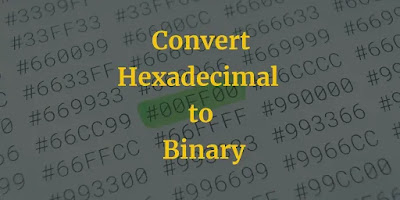# Hexadecimal to binary short conversion methodHexadecimal to Binary

In this post, we will learn the basic steps to convert a hexadecimal number to binary number.

There are two methods to convert hexadecimal to binary, one is the short method and another is long method. Here we will discuss only the steps relating to the short method and the long method of conversion will be covered in later post.

1. The hexadecimal number system has 16 digits starting from 0 to F, where the largest hexadecimal digit is (F)16.
2. Referring to the hexadecimal to binary conversion table, we see that the binary equivalent of the largest hexadecimal digit (F)16 is (1111)2 and minimum of four binary digits(bits) are required to represent the largest hexadecimal digit in the binary system.
3. In order to convert the given hexadecimal number to binary number, we convert each hexadecimal digit into four-bit binary number beginning from the right most hexadecimal digit towards the leftmost digit (MSD).
4. At the end, the four-bit binary numbers are combined to form the single binary number equivalent to the given hexadecimal number.
The above steps are explained with an example for better understanding:

Example 1:Convert hexadecimal number (FA98)16 to binary number (?)2

Step 1: Each digit from the given hexadecimal number is written down leaving small space between each digit as shown below

Hexadecimal Number -> F    A    9    8

Step 2: Referring to the conversion table to find the binary equivalents of each hexadecimal digit.

The binary equivalents are:

F-1111
A-1010
9-1001
8-1000
Write down the four-bit binary numbers below each hexadecimal digit as shown below:

Hexadecimal Number -> F      A      9       8

Binary Number ->  1111 1010  1001  1000

Hence, the binary equivalent of the given hexadecimal number is (1111101010011000)2